Chapter 17. Kinetics

# Concentration–Time Relationships: Integrated Rate Laws

Jessie A. Key

Learning Objectives

• To gain an understanding of graphical methods used to determine rate laws.
• To gain an understanding of half-life with respect to first-order reactions.

An alternate way to determine a rate law is to monitor the concentration of reactants or products in a single trial over a period of time and compare that to what is expected mathematically for a first-, second-, or zero-order reaction.

# First-Order Reactions

We have seen earlier that the rate law of a generic first-order reaction where A → B can be expressed in terms of the reactant concentration: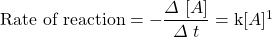This form of the rate law is sometimes referred to as the differential rate law. We can perform a mathematical procedure known as an integration to transform the rate law to another useful form known as the integrated rate law: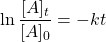where “ln” is the natural logarithm, [A]0 is the initial concentration of A, and [A]t is the concentration of A at another time.

The process of integration is beyond the scope of this textbook, but is covered in most calculus textbooks and courses. The most useful aspect of the integrated rate law is that it can be rearranged to have the general form of a straight line (y = mx + b).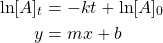Therefore, if we were to graph the natural logarithm of the concentration of a reactant (ln) versus time, a reaction that has a first-order rate law will yield a straight line, while a reaction with any other order will not yield a straight line (Figure 17.7 “Concentration vs. Time, First-Order Reaction”). The slope of the straight line corresponds to the negative rate constant, –k, and the y-intercept corresponds to the natural logarithm of the initial concentration.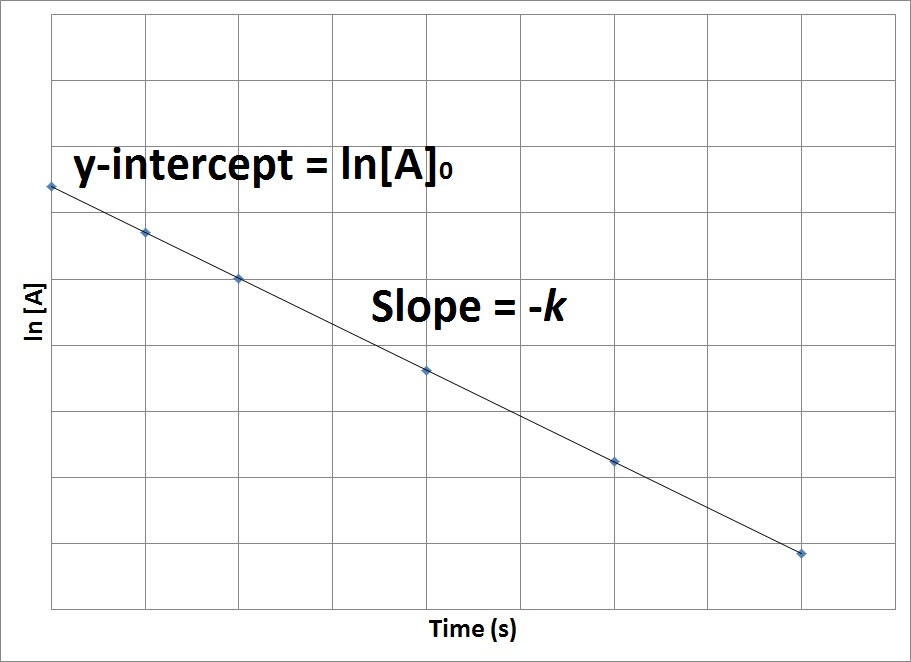Figure 17.7. “Concentration vs. Time, First-Order Reaction.” This graph shows the plot of the natural logarithm of concentration versus time for a first-order reaction.

Example 17.4

The decomposition of a pollutant in water at 15°C occurs with a rate constant of 2.39 y−1, following first-order kinetics. If a local factory spills 6,500 moles of this pollutant into a lake with a volume of 2,500 L, what will the concentration of pollutant be after two years, assuming the lake temperature remains constant at 15°C?

Solution

We are given the rate constant and time and can determine an initial concentration from the number of moles and volume given.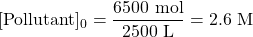We can substitute this data into the integrated rate law of a first-order equation and solve for the concentration after 2.0 years: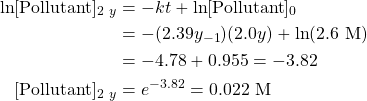# Second-Order Reactions

The rate for second-order reactions depends either on two reactants raised to the first power or a single reactant raised to the second power. We will examine a reaction that is the latter type: C → D. The differential rate law can be written: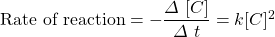The integrated rate law can be written in the form of a straight line as: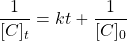Therefore, if the reaction is second order, a plot of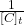versus t will produce a straight line with a slope that corresponds to the rate constant, k, and a y-intercept that corresponds to the inverse of the initial concentration,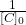(Figure 17.8 “vs. Time, Second-Order Reaction”).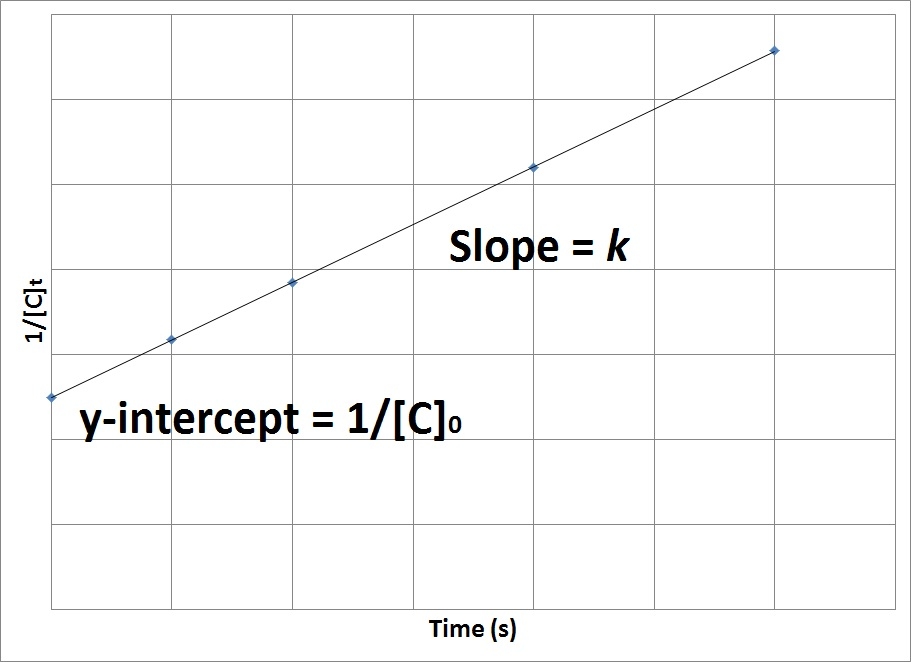Figure 17.8 “ vs. Time, Second-Order Reaction.” The graph shows a plot of versus time for a second-order reaction.

# Zero-Order Reactions

Zero-order reaction rates occur when the rate of reactant disappearance is independent of reactant concentrations. The differential rate law for the hypothetical zero-order reaction E → F could be written as: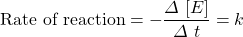The integrated rate law can be written in the form of a straight line as: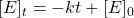Therefore, if the reaction is zero order, a plot of [E] versus t will produce a straight line with a slope that corresponds to the negative of the product of the rate constant and time, −kt, and a y-intercept that corresponds to the initial concentration, [E]0 (Figure 17.9 “Concentration vs. Time, Zero-Order Reaction”).Figure 17.9 “Concentration vs. Time, Zero-Order Reaction.” The graph shows the plot of concentration versus time for a zero-order reaction.

# Graphical Methods for Determining Reaction Order—A Summary

We have just seen that first-, second-, and zero-order reactions all have unique, integrated rate-law equations that allow us to plot them as a straight line (y = mx + b) (Table 17.1 “Integrated Rate Law Summary”). When presented with experimental concentration–time data, we can determine the order by simply plotting the data in different ways to obtain a straight line.

Table 17.1 Integrated Rate Law Summary
Reaction Order Integrated Rate Law Characteristic Kinetic Plot Slope of Kinetic Plot Units of Rate Constant
Zero [E]t = −kt + [E]0 [E] vs. t kt mol L−1s−1
First ln [A]t = −kt + ln [A]0 ln [A] vs. t k s−1
Second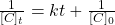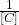vs. t k L mol−1s−1

Example 17.5

The following data were obtained for the reaction 3A → 2B:

 Time, s [A], M 0 5 10 15 20 0.2 0.0282 0.0156 0.0106 0.008

Determine the order of the reaction.

Solution

We can plot the characteristic kinetic plots of zero-, first-, and second-order reactions to determine which will give a straight line.

Time, s [A], mol L−1 ln [A]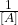, L mol−1
0 0.200 −1.61 5.00
5 0.0282 −3.57 35.5
10 0.0156 −4.16 64.1
15 0.0106 −4.55 94.3
20 0.008 −4.83 125

The reaction is second order since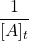versus t gives a straight line.

# Half-Life

The half-life of a reaction,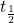, is the duration of time required for the concentration of a reactant to drop to one-half of its initial concentration.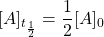Half-life is typically used to describe first-order reactions and serves as a metric to discuss the relative speeds of reactions. A slower reaction will have a longer half-life, while a faster reaction will have a shorter half-life.

To determine the half-life of a first-order reaction, we can manipulate the integrated rate law by substitutingfor t and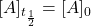for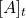, then solve for: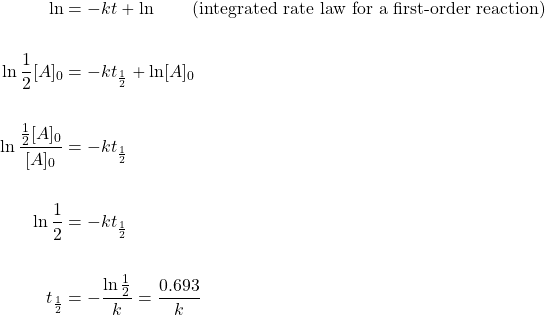Since the half-life equation of a first-order reaction does not include a reactant concentration term, it does not rely on the concentration of reactant present. In other words, a half-life is independent of concentration and remains constant throughout the duration of the reaction. Consequently, plots of kinetic data for first-order reactions exhibit a series of regularly spacedintervals (Figure 17.10 “Generic First-Order Reaction Kinetics Plot”).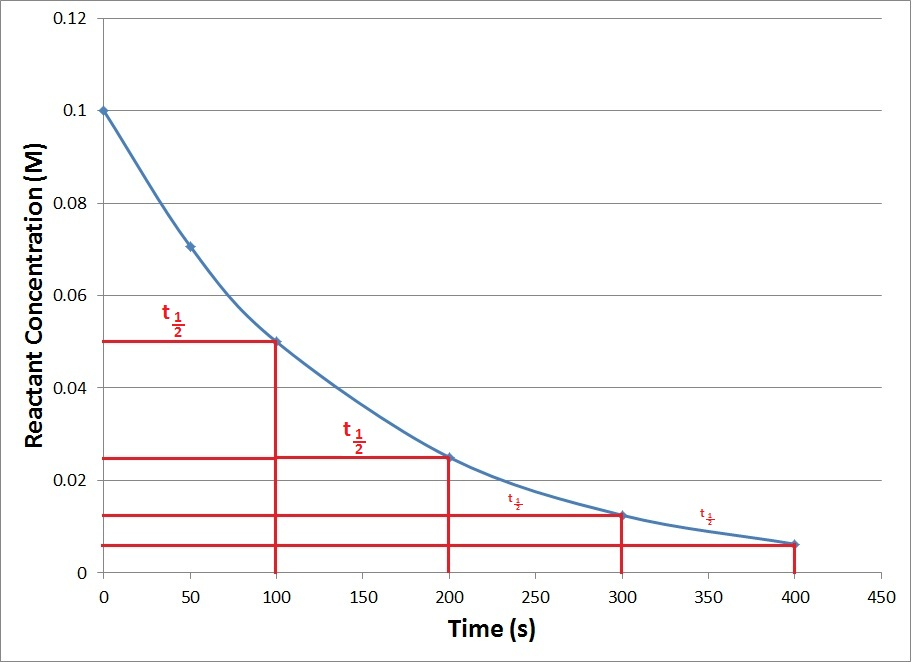Figure 17.10 “Generic First-Order Reaction Kinetics Plot.” This graph shows repeating half-lives on a kinetics plot of a generic first-order reaction.

Example 17.6

A reaction having a first-order rate has a rate constant of 4.00 × 10−3 s−1.

1. Determine the half-life.
2. How long will it take for a sample of reactant at 1.0 M to decrease to 0.25 M?
3. What concentration of the 1.0 M sample of reactant would you expect to be present after it has reacted for 500 s?

Solution

1.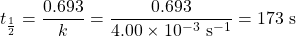2. A simple way to calculate this is to determine how many half-lives it will take to go from 1.00 M to 0.250 M and use the half-life calculated in part 1.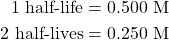Therefore, it will take 2 × 173 s = 346 s.

3. We can use the rate-constant value in the integrated rate law to determine the concentration remaining.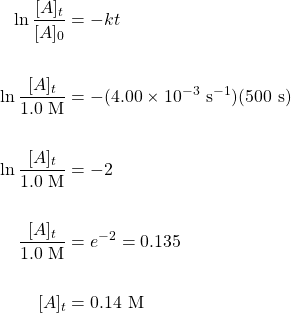Key Takeaways

• The reaction rate may be determined by monitoring the concentration of reactants or products in a single trial over a period of time and comparing it to what is expected mathematically for a first-, second-, or zero-order reaction.
• The half-life of a reaction is the duration of time required for the concentration of a reactant to drop to one-half of its initial concentration.